# filter

Filter disturbances through vector error-correction (VEC) model

## Syntax

``Y = filter(Mdl,Z)``
``````[Y,E] = filter(Mdl,Z)``````
``Tbl2 = filter(Mdl,Tbl1,Presample=Presample)``
``[___] = filter(___,Name,Value)``

## Description

example

````Y = filter(Mdl,Z)` returns the numeric array `Y` containing the multivariate response series, which results from filtering the underlying input numeric array `Z` containing the multivariate disturbance series. The series in `Z` are associated with the model innovations process through the fully specified VEC(p – 1) model `Mdl`.```

example

``````[Y,E] = filter(Mdl,Z)``` returns the numeric array containing the multivariate model innovations series `E`.```

example

````Tbl2 = filter(Mdl,Tbl1,Presample=Presample)` returns the table or timetable `Tbl2` containing the multivariate response series, which results from filtering the underlying multivariate disturbance series in the input table or timetable `Tbl1`. `filter` initializes the response series using the required table or timetable of presample data in `Presample`. Variables in `Tbl1` are associated with the model innovations process through `Mdl`.`filter` selects the variables in `Mdl.SeriesNames` or all variables in `Tbl1`. To select different disturbance variables in `Tbl1` to filter through the model, use the `DisturbanceVariables` name-value argument. `filter` selects the same variables for `Presample` by default, but you can select different variables by using the `PresampleResponseVariables` name-value argument.```

example

````[___] = filter(___,Name,Value)` specifies options using one or more name-value arguments in addition to any of the input argument combinations in previous syntaxes. `filter` returns the output argument combination for the corresponding input arguments. For example, `filter(Mdl,Z,Y0=PS,X=Exo)` filters the numeric array of disturbances `Z` through the VEC(p – 1) model `Mdl`, and specifies the numeric array of presample response data `PS` and the numeric matrix of exogenous predictor data `Exo` for the model regression component.```

## Examples

collapse all

Consider a VEC model for the following seven macroeconomic series. Then, fit the model to the data and filter disturbances through the fitted model. Supply the disturbances as a numeric matrix.

• Gross domestic product (GDP)

• GDP implicit price deflator

• Paid compensation of employees

• Nonfarm business sector hours of all persons

• Effective federal funds rate

• Personal consumption expenditures

• Gross private domestic investment

Suppose that a cointegrating rank of 4 and one short-run term are appropriate, that is, consider a VEC(1) model.

Load the `Data_USEconVECModel` data set.

`load Data_USEconVECModel`

For more information on the data set and variables, enter `Description` at the command line.

Determine whether the data needs to be preprocessed by plotting the series on separate plots.

```figure tiledlayout(2,2) nexttile plot(FRED.Time,FRED.GDP) title("Gross Domestic Product") ylabel("Index") xlabel("Date") nexttile plot(FRED.Time,FRED.GDPDEF) title("GDP Deflator") ylabel("Index") xlabel("Date") nexttile plot(FRED.Time,FRED.COE) title("Paid Compensation of Employees") ylabel("Billions of \$") xlabel("Date") nexttile plot(FRED.Time,FRED.HOANBS) title("Nonfarm Business Sector Hours") ylabel("Index") xlabel("Date")```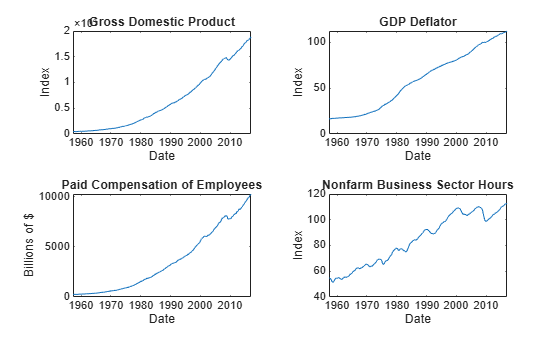```figure tiledlayout(2,2) nexttile plot(FRED.Time,FRED.FEDFUNDS) title("Federal Funds Rate") ylabel("Percent") xlabel("Date") nexttile plot(FRED.Time,FRED.PCEC) title("Consumption Expenditures") ylabel("Billions of \$") xlabel("Date") nexttile plot(FRED.Time,FRED.GPDI) title("Gross Private Domestic Investment") ylabel("Billions of \$") xlabel("Date")```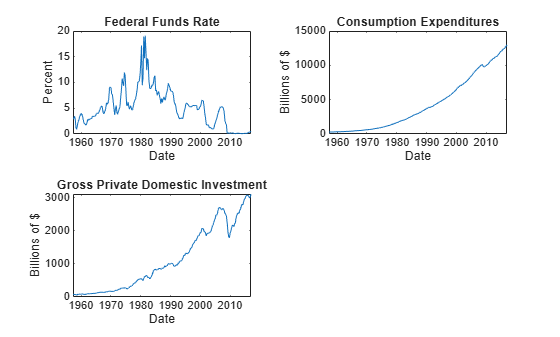Stabilize all series, except the federal funds rate, by applying the log transform. Scale the resulting series by 100 so that all series are on the same scale.

```FRED.GDP = 100*log(FRED.GDP); FRED.GDPDEF = 100*log(FRED.GDPDEF); FRED.COE = 100*log(FRED.COE); FRED.HOANBS = 100*log(FRED.HOANBS); FRED.PCEC = 100*log(FRED.PCEC); FRED.GPDI = 100*log(FRED.GPDI);```

Create a VEC(1) model using the shorthand syntax. Specify the variable names.

```Mdl = vecm(7,4,1); Mdl.SeriesNames = FRED.Properties.VariableNames```
```Mdl = vecm with properties: Description: "7-Dimensional Rank = 4 VEC(1) Model with Linear Time Trend" SeriesNames: "GDP" "GDPDEF" "COE" ... and 4 more NumSeries: 7 Rank: 4 P: 2 Constant: [7×1 vector of NaNs] Adjustment: [7×4 matrix of NaNs] Cointegration: [7×4 matrix of NaNs] Impact: [7×7 matrix of NaNs] CointegrationConstant: [4×1 vector of NaNs] CointegrationTrend: [4×1 vector of NaNs] ShortRun: {7×7 matrix of NaNs} at lag  Trend: [7×1 vector of NaNs] Beta: [7×0 matrix] Covariance: [7×7 matrix of NaNs] ```

`Mdl` is a `vecm` model object. All properties containing `NaN` values correspond to parameters to be estimated given data.

Estimate the model using the entire data set and the default options. By default, `estimate` uses the first p = 2 observations as presample data.

`EstMdl = estimate(Mdl,FRED.Variables)`
```EstMdl = vecm with properties: Description: "7-Dimensional Rank = 4 VEC(1) Model" SeriesNames: "GDP" "GDPDEF" "COE" ... and 4 more NumSeries: 7 Rank: 4 P: 2 Constant: [14.1329 8.77841 -7.20359 ... and 4 more]' Adjustment: [7×4 matrix] Cointegration: [7×4 matrix] Impact: [7×7 matrix] CointegrationConstant: [-28.6082 109.555 -77.0912 ... and 1 more]' CointegrationTrend: [4×1 vector of zeros] ShortRun: {7×7 matrix} at lag  Trend: [7×1 vector of zeros] Beta: [7×0 matrix] Covariance: [7×7 matrix] ```

`EstMdl` is an estimated `vecm` model object. It is fully specified because all parameters have known values. By default, `estimate` imposes the constraints of the H1 Johansen VEC model form by removing the cointegrating trend and linear trend terms from the model. Parameter exclusion from estimation is equivalent to imposing equality constraints to zero.

Generate a `numobs`-by-7 series of random Gaussian distributed values, where `numobs` is the number of observations in the data minus p.

```numobs = size(FRED,1) - Mdl.P; rng(1) % For reproducibility Z = randn(numobs,Mdl.NumSeries);```

To simulate responses, filter the disturbances through the estimated model. Specify the first p = 2 observations as presample data.

`Y = filter(EstMdl,Z,Y0=FRED{1:2,:});`

`Y` is a 238-by-7 matrix of simulated responses. Columns correspond to the variable names in `EstMdl.SeriesNames`.

Plot the simulated and true responses.

```figure tiledlayout(2,2) nexttile plot(FRED.Time(3:end),[FRED.GDP(3:end) Y(:,1)]) title("Gross Domestic Product") ylabel("Index (scaled)") xlabel("Date") legend("Simulation","True","Location","Best") nexttile plot(FRED.Time(3:end),[FRED.GDPDEF(3:end) Y(:,2)]) title("GDP Deflator") ylabel("Index (scaled)") xlabel("Date") legend("Simulation","True","Location","Best") nexttile plot(FRED.Time(3:end),[FRED.COE(3:end) Y(:,3)]) title("Paid Compensation of Employees") ylabel("Billions of \$ (scaled)") xlabel("Date") legend("Simulation","True","Location","Best") nexttile plot(FRED.Time(3:end),[FRED.HOANBS(3:end) Y(:,4)]) title("Nonfarm Business Sector Hours") ylabel("Index (scaled)") xlabel("Date") legend("Simulation","True","Location","Best")```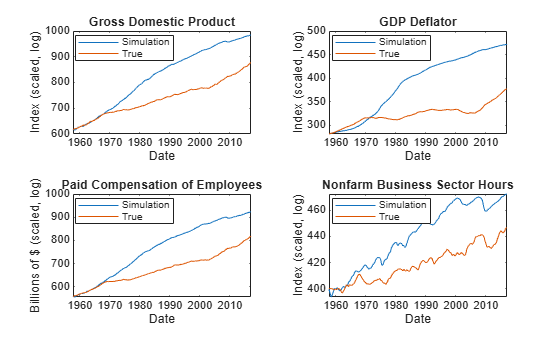```figure tiledlayout(2,2) nexttile plot(FRED.Time(3:end),[FRED.FEDFUNDS(3:end) Y(:,5)]) title("Federal Funds Rate") ylabel("Percent") xlabel("Date") nexttile plot(FRED.Time(3:end),[FRED.PCEC(3:end) Y(:,6)]) title("Consumption Expenditures") ylabel("Billions of \$ (scaled)") xlabel("Date") nexttile plot(FRED.Time(3:end),[FRED.GPDI(3:end) Y(:,7)]) title("Gross Private Domestic Investment") ylabel("Billions of \$ (scaled)") xlabel("Date")```Consider this VEC(1) model for three hypothetical response series.

`$\begin{array}{rcl}\Delta {y}_{t}& =& c+A{B}^{\prime }{y}_{t-1}+{\Phi }_{1}\Delta {y}_{t-1}+{\epsilon }_{t}\\ & =& \\ & =& \left[\begin{array}{c}-1\\ -3\\ -30\end{array}\right]+\left[\begin{array}{cc}-0.3& 0.3\\ -0.2& 0.1\\ -1& 0\end{array}\right]\left[\begin{array}{ccc}0.1& -0.2& 0.2\\ -0.7& 0.5& 0.2\end{array}\right]{y}_{t-1}+\left[\begin{array}{ccc}0& 0.1& 0.2\\ 0.2& -0.2& 0\\ 0.7& -0.2& 0.3\end{array}\right]\Delta {y}_{t-1}+{\epsilon }_{t}.\end{array}$`

The innovations are multivariate Gaussian with a mean of 0 and the covariance matrix

`$\Sigma =\left[\begin{array}{ccc}1.3& 0.4& 1.6\\ 0.4& 0.6& 0.7\\ 1.6& 0.7& 5\end{array}\right].$`

Create variables for the parameter values.

```Adjustment = [-0.3 0.3; -0.2 0.1; -1 0]; Cointegration = [0.1 -0.7; -0.2 0.5; 0.2 0.2]; ShortRun = {[0. 0.1 0.2; 0.2 -0.2 0; 0.7 -0.2 0.3]}; Constant = [-1; -3; -30]; Trend = [0; 0; 0]; Covariance = [1.3 0.4 1.6; 0.4 0.6 0.7; 1.6 0.7 5];```

Create a `vecm` model object representing the VEC(1) model using the appropriate name-value pair arguments.

```Mdl = vecm('Adjustment',Adjustment,'Cointegration',Cointegration,... 'Constant',Constant,'ShortRun',ShortRun,'Trend',Trend,... 'Covariance',Covariance)```
```Mdl = vecm with properties: Description: "3-Dimensional Rank = 2 VEC(1) Model" SeriesNames: "Y1" "Y2" "Y3" NumSeries: 3 Rank: 2 P: 2 Constant: [-1 -3 -30]' Adjustment: [3×2 matrix] Cointegration: [3×2 matrix] Impact: [3×3 matrix] CointegrationConstant: [2×1 vector of NaNs] CointegrationTrend: [2×1 vector of NaNs] ShortRun: {3×3 matrix} at lag  Trend: [3×1 vector of zeros] Beta: [3×0 matrix] Covariance: [3×3 matrix] ```

`Mdl` is, effectively, a fully specified `vecm` model object. That is, the cointegration constant and linear trend are unknown. However, they are not needed for simulating observations or forecasting, given that the overall constant and trend parameters are known.

Generate 1000 paths of 100 observations from a 3-D Gaussian distribution. `numobs` is the number of observations in the data without any missing values.

```numobs = 100; numpaths = 1000; rng(1); Z = randn(numobs,Mdl.NumSeries,numpaths);```

Filter the disturbances through the estimated model. Return the innovations (scaled disturbances).

`[Y,E] = filter(Mdl,Z);`

`Y` and `E` are 100-by-3-by-1000 matrices of filtered responses and scaled disturbances, respectively.

For each time point, compute the mean vector of the filtered responses among all paths.

`MeanFilt = mean(Y,3);`

`MeanFilt` is a 100-by-3 matrix containing the average of the filtered responses at each time point.

Plot the filtered responses and their averages.

```figure; for j = 1:Mdl.NumSeries subplot(2,2,j) plot(squeeze(Y(:,j,:)),'Color',[0.8,0.8,0.8]) title(Mdl.SeriesNames{j}); hold on plot(MeanFilt(:,j)); xlabel('Time index') hold off end```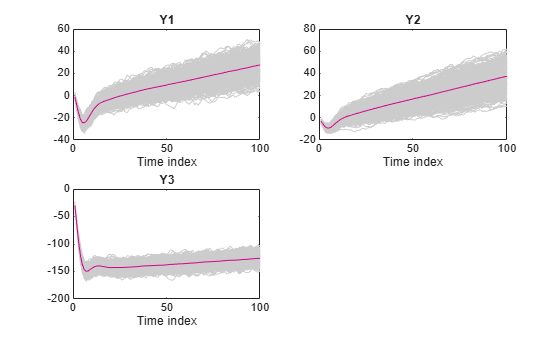Fit a VEC(1) model to seven macroeconomic series. Then, simulate responses by filtering multiple random paths of Gaussian distributed disturbances through the estimated model. Supply the disturbances in a timetable. This example is based on Fit VEC(1) Model to Matrix of Response Data.

Load and Preprocess Data

Load the `Data_USEconVECModel` data set.

```load Data_USEconVECModel head(FRED)```
``` Time GDP GDPDEF COE HOANBS FEDFUNDS PCEC GPDI ___________ _____ ______ _____ ______ ________ _____ ____ 31-Mar-1957 470.6 16.485 260.6 54.756 2.96 282.3 77.7 30-Jun-1957 472.8 16.601 262.5 54.639 3 284.6 77.9 30-Sep-1957 480.3 16.701 265.1 54.375 3.47 289.2 79.3 31-Dec-1957 475.7 16.711 263.7 53.249 2.98 290.8 71 31-Mar-1958 468.4 16.892 260.2 52.043 1.2 290.3 66.7 30-Jun-1958 472.8 16.94 259.9 51.297 0.93 293.2 65.1 30-Sep-1958 486.7 17.043 267.7 51.908 1.76 298.3 72 31-Dec-1958 500.4 17.123 272.7 52.683 2.42 302.2 80 ```

Stabilize all series, except the federal funds rate, by applying the log transform. Scale the resulting series by 100 so that all series are on the same scale.

```FRED.GDP = 100*log(FRED.GDP); FRED.GDPDEF = 100*log(FRED.GDPDEF); FRED.COE = 100*log(FRED.COE); FRED.HOANBS = 100*log(FRED.HOANBS); FRED.PCEC = 100*log(FRED.PCEC); FRED.GPDI = 100*log(FRED.GPDI); numobs = height(FRED)```
```numobs = 240 ```

Prepare Timetable for Estimation

When you plan to supply a timetable directly to estimate, you must ensure it has all the following characteristics:

• All selected response variables are numeric and do not contain any missing values.

• The timestamps in the `Time` variable are regular, and they are ascending or descending.

Remove all missing values from the table.

```DTT = rmmissing(FRED); numobs = height(DTT)```
```numobs = 240 ```

`DTT` does not contain any missing values.

Determine whether the sampling timestamps have a regular frequency and are sorted.

`areTimestampsRegular = isregular(DTT,"quarters")`
```areTimestampsRegular = logical 0 ```
`areTimestampsSorted = issorted(DTT.Time)`
```areTimestampsSorted = logical 1 ```

`areTimestampsRegular = 0` indicates that the timestamps of DTT are irregular. `areTimestampsSorted = 1` indicates that the timestamps are sorted. Macroeconomic series in this example are timestamped at the end of the month. This quality induces an irregularly measured series.

Remedy the time irregularity by shifting all dates to the first day of the quarter.

```dt = DTT.Time; dt = dateshift(dt,"start","quarter"); DTT.Time = dt; areTimestampsRegular = isregular(DTT,"quarters")```
```areTimestampsRegular = logical 1 ```

`DTT` is regular with respect to time.

Fit Model to Data

Create a VEC(1) model using the shorthand syntax. Specify the variable names.

```Mdl = vecm(7,4,1); Mdl.SeriesNames = string(FRED.Properties.VariableNames);```

Estimate the model. Pass the entire timetable `DTT`. By default, `estimate` selects the response variables in `Mdl.SeriesNames` to fit to the model. Alternatively, you can use the `ResponseVariables` name-value argument.

`EstMdl = estimate(Mdl,DTT);`

Simulate Paths of Disturbances

Generate a `numobs`-by-`numseries`-by-`numpaths` array of independent random Gaussian distributed values, where `numobs` is the number of observations in the data, `numseries` the number of response series 7, and `numpaths` is 100. Add the matrices of simulated paths into the data set `DTT`.

```rng(1) % For reproducibility numobs = height(DTT); numseries = EstMdl.NumSeries; numpaths = 100; Z = mvnrnd(zeros(numseries,1),eye(numseries),numobs*numpaths); Z = reshape(Z,numobs,numseries,numpaths); for j = 1:numseries DTT = addvars(DTT,squeeze(Z(:,j,:)), ... NewVariableNames="Z_" + EstMdl.SeriesNames{j}); end head(DTT)```
``` Time GDP GDPDEF COE HOANBS FEDFUNDS PCEC GPDI Z_GDP Z_GDPDEF Z_COE Z_HOANBS Z_FEDFUNDS Z_PCEC Z_GPDI ___________ ______ ______ ______ ______ ________ ______ ______ ____________ ____________ ____________ ____________ ____________ ____________ ____________ 01-Jan-1957 615.4 280.25 556.3 400.29 2.96 564.3 435.29 1x100 double 1x100 double 1x100 double 1x100 double 1x100 double 1x100 double 1x100 double 01-Apr-1957 615.87 280.95 557.03 400.07 3 565.11 435.54 1x100 double 1x100 double 1x100 double 1x100 double 1x100 double 1x100 double 1x100 double 01-Jul-1957 617.44 281.55 558.01 399.59 3.47 566.71 437.32 1x100 double 1x100 double 1x100 double 1x100 double 1x100 double 1x100 double 1x100 double 01-Oct-1957 616.48 281.61 557.48 397.5 2.98 567.26 426.27 1x100 double 1x100 double 1x100 double 1x100 double 1x100 double 1x100 double 1x100 double 01-Jan-1958 614.93 282.68 556.15 395.21 1.2 567.09 420.02 1x100 double 1x100 double 1x100 double 1x100 double 1x100 double 1x100 double 1x100 double 01-Apr-1958 615.87 282.97 556.03 393.76 0.93 568.09 417.59 1x100 double 1x100 double 1x100 double 1x100 double 1x100 double 1x100 double 1x100 double 01-Jul-1958 618.76 283.57 558.99 394.95 1.76 569.81 427.67 1x100 double 1x100 double 1x100 double 1x100 double 1x100 double 1x100 double 1x100 double 01-Oct-1958 621.54 284.04 560.84 396.43 2.42 571.11 438.2 1x100 double 1x100 double 1x100 double 1x100 double 1x100 double 1x100 double 1x100 double ```

Filter Disturbances Through Model

When you filter disturbances by using a timetable, `filter` requires a presample. Split the timetable into presample and in-sample data sets. The presample data is the initial `EstMdl.P` observations, and the in-sample data set contains the remaining observations.

```Presample = DTT(1:EstMdl.P,:); InSample = DTT((EstMdl.P + 1):end,:);```

Simulate response paths by filtering the in-sample disturbances through the estimated model. Specify the variable names of the disturbance series, the presample data, and the response variable names in the presample.

```dnames = string(DTT.Properties.VariableNames); idx = startsWith(dnames,"Z_"); dnames = dnames(idx); Tbl2 = filter(EstMdl,InSample,DisturbanceVariables=dnames, ... Presample=Presample,PresampleResponseVariables=EstMdl.SeriesNames); size(Tbl2)```
```ans = 1×2 238 28 ```
`head(Tbl2)`
``` Time GDP GDPDEF COE HOANBS FEDFUNDS PCEC GPDI Z_GDP Z_GDPDEF Z_COE Z_HOANBS Z_FEDFUNDS Z_PCEC Z_GPDI GDP_Responses GDPDEF_Responses COE_Responses HOANBS_Responses FEDFUNDS_Responses PCEC_Responses GPDI_Responses GDP_Innovations GDPDEF_Innovations COE_Innovations HOANBS_Innovations FEDFUNDS_Innovations PCEC_Innovations GPDI_Innovations ___________ ______ ______ ______ ______ ________ ______ ______ ____________ ____________ ____________ ____________ ____________ ____________ ____________ _____________ ________________ _____________ ________________ __________________ ______________ ______________ _______________ __________________ _______________ __________________ ____________________ ________________ ________________ 01-Jul-1957 617.44 281.55 558.01 399.59 3.47 566.71 437.32 1x100 double 1x100 double 1x100 double 1x100 double 1x100 double 1x100 double 1x100 double 1x100 double 1x100 double 1x100 double 1x100 double 1x100 double 1x100 double 1x100 double 1x100 double 1x100 double 1x100 double 1x100 double 1x100 double 1x100 double 1x100 double 01-Oct-1957 616.48 281.61 557.48 397.5 2.98 567.26 426.27 1x100 double 1x100 double 1x100 double 1x100 double 1x100 double 1x100 double 1x100 double 1x100 double 1x100 double 1x100 double 1x100 double 1x100 double 1x100 double 1x100 double 1x100 double 1x100 double 1x100 double 1x100 double 1x100 double 1x100 double 1x100 double 01-Jan-1958 614.93 282.68 556.15 395.21 1.2 567.09 420.02 1x100 double 1x100 double 1x100 double 1x100 double 1x100 double 1x100 double 1x100 double 1x100 double 1x100 double 1x100 double 1x100 double 1x100 double 1x100 double 1x100 double 1x100 double 1x100 double 1x100 double 1x100 double 1x100 double 1x100 double 1x100 double 01-Apr-1958 615.87 282.97 556.03 393.76 0.93 568.09 417.59 1x100 double 1x100 double 1x100 double 1x100 double 1x100 double 1x100 double 1x100 double 1x100 double 1x100 double 1x100 double 1x100 double 1x100 double 1x100 double 1x100 double 1x100 double 1x100 double 1x100 double 1x100 double 1x100 double 1x100 double 1x100 double 01-Jul-1958 618.76 283.57 558.99 394.95 1.76 569.81 427.67 1x100 double 1x100 double 1x100 double 1x100 double 1x100 double 1x100 double 1x100 double 1x100 double 1x100 double 1x100 double 1x100 double 1x100 double 1x100 double 1x100 double 1x100 double 1x100 double 1x100 double 1x100 double 1x100 double 1x100 double 1x100 double 01-Oct-1958 621.54 284.04 560.84 396.43 2.42 571.11 438.2 1x100 double 1x100 double 1x100 double 1x100 double 1x100 double 1x100 double 1x100 double 1x100 double 1x100 double 1x100 double 1x100 double 1x100 double 1x100 double 1x100 double 1x100 double 1x100 double 1x100 double 1x100 double 1x100 double 1x100 double 1x100 double 01-Jan-1959 623.66 284.31 563.55 398.35 2.8 573.62 442.12 1x100 double 1x100 double 1x100 double 1x100 double 1x100 double 1x100 double 1x100 double 1x100 double 1x100 double 1x100 double 1x100 double 1x100 double 1x100 double 1x100 double 1x100 double 1x100 double 1x100 double 1x100 double 1x100 double 1x100 double 1x100 double 01-Apr-1959 626.19 284.46 565.91 400.24 3.39 575.54 449.31 1x100 double 1x100 double 1x100 double 1x100 double 1x100 double 1x100 double 1x100 double 1x100 double 1x100 double 1x100 double 1x100 double 1x100 double 1x100 double 1x100 double 1x100 double 1x100 double 1x100 double 1x100 double 1x100 double 1x100 double 1x100 double ```

`Tbl2` is a 238-by-2 matrix of in-sample data, paths of simulated disturbances, paths of filtered responses (variables names appended with `_Responses`, and paths of innovations (variables with name appended with `_Innovations`).

```rnames = string(Tbl2.Properties.VariableNames); idx = endsWith(rnames,"_Responses"); rnames = rnames(idx); figure tiledlayout(2,2) for j = 1:4 nexttile p1 = plot(Tbl2.Time,Tbl2{:,rnames(j)},Color=[0.5 0.5 0.5]); hold on p2 = plot(Tbl2.Time,Tbl2{:,Mdl.SeriesNames(j)},LineWidth=2); title(Mdl.SeriesNames(j)) xlabel("Date") legend([p1(1) p2],["Simulated" "Observed"]) end```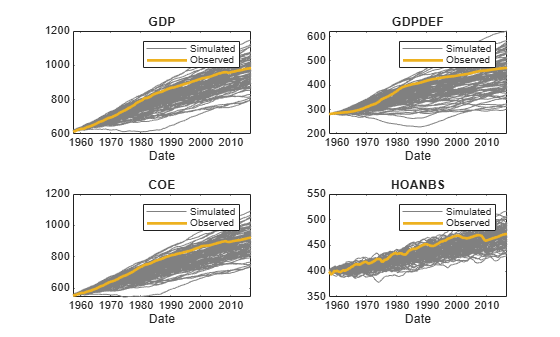```figure tiledlayout(2,2) for j = 5:7 nexttile p1 = plot(Tbl2.Time,Tbl2{:,rnames(j)},Color=[0.5 0.5 0.5]); hold on p2 = plot(Tbl2.Time,Tbl2{:,Mdl.SeriesNames(j)},LineWidth=2); title(Mdl.SeriesNames(j)) xlabel("Date") legend([p1(1) p2],["Simulated" "Observed"]) end```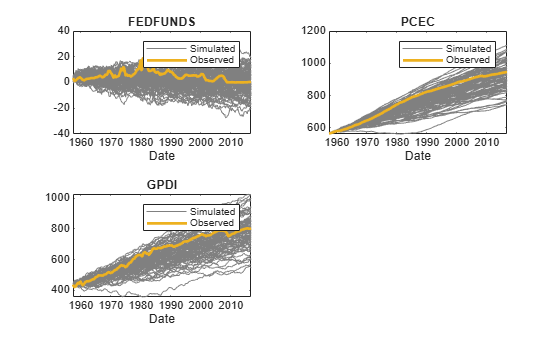## Input Arguments

collapse all

VEC model, specified as a `vecm` model object created by `vecm` or `estimate`. `Mdl` must be fully specified.

Underlying multivariate disturbance series zt associated with the model innovations process εt, specified as a `numobs`-by-`numseries` numeric matrix or a `numobs`-by-`numseries`-by-`numpaths` numeric array.

`numobs` is the sample size. `numseries` is the number of disturbance series (`Mdl.NumSeries`). `numpaths` is the number of disturbance paths.

Rows correspond to sampling times, and the last row contains the latest set of disturbances.

Columns correspond to individual disturbance series for response variables.

Pages correspond to separate, independent paths. For a numeric matrix, `Z` is a single `numseries`-dimensional path of disturbance series. For a 3-D array, each page of `Z` represents a separate `numseries`-dimensional path. Among all pages, disturbances in corresponding rows occur at the same time.

The `Scale` name-value argument specifies whether to scale the disturbances before `filter` filters them through `Mdl`. For more details, see `Scale`.

Data Types: `double`

Time series data containing observed disturbance variables zt, associated with the model innovations process εt, or predictor variables xt, specified as a table or timetable with `numvars` variables and `numobs` rows. You can optionally select `numseries` disturbance variables or `numpreds` predictor variables by using the `DisturbanceVariables` or `PredictorVariables` name-value arguments, respectively.

Each selected disturbance variable is a `numobs`-by-`numpaths` numeric matrix, and each predictor variable is a numeric vector. Each row is an observation, and measurements in each row occur simultaneously.

Each path (column) within a particular variable are independent, but path `j` of all variables correspond, for `j` = 1,…,`numpaths`.

If `Tbl1` is a timetable, it must represent a sample with a regular datetime time step (see `isregular`), and the datetime vector `Tbl1.Time` must be strictly ascending or descending.

If `Tbl1` is a table, the following conditions hold:

• The last row contains the latest observation.

• `Tbl1.Properties.RowsNames` must be empty.

The `Scale` name-value argument specifies whether to scale the disturbances before `filter` filters them through `Mdl`. For more details, see `Scale`.

Presample data that provides initial values for the model `Mdl`, specified as a table or timetable, the same type as `Tbl1`, with `numprevars` variables and `numpreobs` rows. `Presample` is required when you supply a table or timetable of data `Tbl1`.

Each row is a presample observation, and measurements in each row, among all paths, occur simultaneously. `numpreobs` must be at least `Mdl.P`. If you supply more rows than necessary, `filter` uses the latest `Mdl.P` observations only.

Each variable is a `numpreobs`-by-`numprepaths` numeric matrix. Variables correspond to the response series associated with the respective disturbance in `Tbl1`. To control presample variable selection, see the optional `PresampleResponseVariables` name-value argument.

For each variable, columns are separate, independent paths.

• If variables are vectors, `filter` applies them to each path in `Tbl1` to produce the filtered responses in `Tbl2`. Therefore, all paths of filtered responses derive from common initial conditions.

• Otherwise, for each variable `Vark` and each path `j`, `filter` applies `Presample.Vark(:,j)` to produce `Tbl2.Vark(:,j)`. Variables must have at least `numpaths` columns, and `filter` uses only the first `numpaths` columns.

If `Presample` is a timetable, all the following conditions must be true:

• `Presample` must represent a sample with a regular datetime time step (see `isregular`).

• The inputs `Tbl1` and `Presample` must be consistent in time such that `Presample` immediately precedes `Tbl1` with respect to the sampling frequency and order.

• The datetime vector of sample timestamps `Presample.Time` must be ascending or descending.

If `Presample` is a table, the following conditions hold:

• The last row contains the latest presample observation.

• `Presample.Properties.RowsNames` must be empty.

### Name-Value Arguments

Specify optional pairs of arguments as `Name1=Value1,...,NameN=ValueN`, where `Name` is the argument name and `Value` is the corresponding value. Name-value arguments must appear after other arguments, but the order of the pairs does not matter.

Before R2021a, use commas to separate each name and value, and enclose `Name` in quotes.

Example: `filter(Mdl,Z,Y0=PS,X=Exo)` filters the numeric array of disturbances `Z` through the VEC(p – 1) model `Mdl`, and specifies the numeric array of presample response data `PS` and the numeric matrix of exogenous predictor data `Exo` for the model regression component.

Variables to select from `Tbl1` to treat as disturbance variables zt, specified as one of the following data types:

• String vector or cell vector of character vectors containing `numseries` variable names in `Tbl1.Properties.VariableNames`

• A length `numseries` vector of unique indices (integers) of variables to select from `Tbl1.Properties.VariableNames`

• A length `numvars` logical vector, where ```DisturbanceVariables(j) = true``` selects variable `j` from `Tbl1.Properties.VariableNames`, and `sum(DisturbanceVariables)` is `numseries`

The selected variables must be numeric vectors (single path) or matrices (columns represent multiple independent paths) of the same width, and cannot contain missing values (`NaN`).

If the number of variables in `Tbl1` matches `Mdl.NumSeries`, the default specifies all variables in `Tbl1`. If the number of variables in `Tbl1` exceeds `Mdl.NumSeries`, the default matches variables in `Tbl1` to names in `Mdl.SeriesNames`.

Example: `DisturbanceVariables=["GDP" "CPI"]`

Example: `DisturbanceVariables=[true false true false]` or `DisturbanceVariable=[1 3]` selects the first and third table variables as the disturbance variables.

Data Types: `double` | `logical` | `char` | `cell` | `string`

Presample responses that provide initial values for the model `Mdl`, specified as a `numpreobs`-by-`numseries` numeric matrix or a `numpreobs`-by-`numseries`-by-`numprepaths` numeric array. Use `Y0` only when you supply a numeric array of disturbance data `Z`.

`numpreobs` is the number of presample observations. `numprepaths` is the number of presample response paths.

Each row is a presample observation, and measurements in each row, among all pages, occur simultaneously. The last row contains the latest presample observation. `Y0` must have at least `Mdl.P` rows. If you supply more rows than necessary, `filter` uses the latest `Mdl.P` observations only.

Each column corresponds to the response series associated with the respective disturbance in `Z`.

Pages correspond to separate, independent paths.

• If `Y0` is a matrix, `filter` applies it to each path (page) to produce the filtered responses `Y`. Therefore, all paths in `Y` derive from common initial conditions.

• Otherwise, `filter` applies `Y0(:,:,j)` to produce `Y(:,:,j)`. `Y0` must have at least `numpaths` pages, and `filter` uses only the first `numpaths` pages.

By default, `filter` sets any necessary presample observations.

• For stationary VAR processes without regression components, `filter` uses the unconditional mean $\mu ={\Phi }^{-1}\left(L\right)c.$

• For nonstationary processes or models containing a regression component, `filter` sets presample observations to an array composed of zeros.

Data Types: `double`

Variables to select from `Presample` to use for presample data, specified as one of the following data types:

• String vector or cell vector of character vectors containing `numseries` variable names in `Presample.Properties.VariableNames`

• A length `numseries` vector of unique indices (integers) of variables to select from `Presample.Properties.VariableNames`

• A length `numvars` logical vector, where ```PresampleResponseVariables(j) = true ``` selects variable `j` from `Presample.Properties.VariableNames`, and `sum(PresampleResponseVariables)` is `numseries`

The selected variables must be numeric vectors (single path) or matrices (columns represent multiple independent paths) of the same width, and cannot contain missing values (`NaN`).

`PresampleResponseNames` does not need to contain the same names as in `Tbl1`; `filter` uses the data in selected variable `PresampleResponseVariables(j)` as a presample for the response variable corresponding to `DisturbanceVariables(j)`.

The default specifies the same response variables as those selected from `Tbl1`, see `DisturbanceVariables`.

Example: `PresampleResponseVariables=["GDP" "CPI"]`

Example: `PresampleResponseVariables=[true false true false]` or `PresampleResponseVariable=[1 3]` selects the first and third table variables for presample data.

Data Types: `double` | `logical` | `char` | `cell` | `string`

Predictor data xt for the regression component in the model, specified as a numeric matrix containing `numpreds` columns. Use `X` only when you supply a numeric array of disturbance data `Z`.

`numpreds` is the number of predictor variables (`size(Mdl.Beta,2)`).

Each row corresponds to an observation, and measurements in each row occur simultaneously. The last row contains the latest observation. `X` must have at least as many observations as `Z`. If you supply more rows than necessary, `filter` uses only the latest observations. `filter` does not use the regression component in the presample period.

Each column is an individual predictor variable. All predictor variables are present in the regression component of each response equation.

`filter` applies `X` to each path (page) in `Z`; that is, `X` represents one path of observed predictors.

By default, `filter` excludes the regression component, regardless of its presence in `Mdl`.

Data Types: `double`

Variables to select from `Tbl1` to treat as exogenous predictor variables xt, specified as one of the following data types:

• String vector or cell vector of character vectors containing `numpreds` variable names in `Tbl1.Properties.VariableNames`

• A length `numpreds` vector of unique indices (integers) of variables to select from `Tbl1.Properties.VariableNames`

• A length `numvars` logical vector, where `PredictorVariables(j) = true ` selects variable `j` from `Tbl1.Properties.VariableNames`, and `sum(PredictorVariables)` is `numpreds`

The selected variables must be numeric vectors and cannot contain missing values (`NaN`).

By default, `filter` excludes the regression component, regardless of its presence in `Mdl`.

Example: `PredictorVariables=["M1SL" "TB3MS" "UNRATE"]`

Example: `PredictorVariables=[true false true false]` or `PredictorVariable=[1 3]` selects the first and third table variables as the response variables.

Data Types: `double` | `logical` | `char` | `cell` | `string`

Flag indicating whether to scale disturbances by the lower triangular Cholesky factor of the model covariance matrix, specified as a value in this table. In the table:

ValueDescription
`true``E(:,:,j)` = `L*Z(:,:,j)`, where `L` = `chol(Mdl.Covariance,"lower")`.
`false`No scale, `E(:,:,j)` = `Z(:,:,j)`

For each page `j` = 1,...,`numpaths`, `filter` filters the `numobs`-by-`numseries` matrix of innovations `E(:,:,j)` through the VAR(p) model `Mdl` using the specified scale.

Example: `Scale=false`

Data Types: `logical`

Note

• `NaN` values in `Z`, `Y0`, and `X` indicate missing values. `filter` removes missing values from the data by list-wise deletion.

1. If `Z` is a 3-D array, then `filter` horizontally concatenates the pages of `Z` to form a `numobs`-by-`numpaths*numseries` matrix.

2. If a regression component is present, then `filter` horizontally concatenates `X` to `Z` to form a `numobs`-by-```(numpaths*numseries + numpreds)``` matrix. `filter` assumes that the last rows of each series occur at the same time.

3. `filter` removes any row that contains at least one `NaN` from the concatenated data.

4. `filter` applies steps 1 and 3 to the presample paths in `Y0`.

This process ensures that the filtered responses and innovations of each path are the same size and are based on the same observation times. In the case of missing observations, the results obtained from multiple paths of `Z` can differ from the results obtained from each path individually.

This data reduction reduces the effective sample size.

• `filter` issues an error when any table or timetable input contains missing values.

## Output Arguments

collapse all

Filtered multivariate response series yt, returned as a `numobs`-by-`numseries` numeric matrix or a `numobs`-by-`numseries`-by-`numpaths` numeric array. `Y` represents the continuation of the presample responses in `Y0`.

`filter` returns `Y` only when you supply the input `Z`.

Multivariate model innovations series εt, returned as a `numobs`-by-`numseries` numeric matrix or a `numobs`-by-`numseries`-by-`numpaths` numeric array. For details on the value of `E`, see `Scale`.

`filter` returns `E` only when you supply the input `Z`.

Multivariate filtered response yt and innovation series εt, returned as a table or timetable, the same data type as `Tbl1`. `filter` returns `Tbl2` only when you supply the input `Tbl1`.

`Tbl2` contains the following variables:

• The filtered response variables yt. Each filtered response variable is a `numobs`-by-`numpaths` numeric matrix, with rows representing observations and columns representing independent paths, each corresponding to the input observations and paths in `Tbl1`. `filter` names the filtered response for disturbance variable `DisturbanceJ` in `Tbl1` `DisturbanceJ_Responses`. For example, if one of the selected disturbance variables in `Tbl1` to filter is `GDP`, `Tbl2` contains a variable for the corresponding filtered responses with the name `GDP_Responses`.

• The innovation variables εt. Each innovation variable is a `numobs`-by-`numpaths` numeric matrix, with rows representing observations and columns representing independent paths, each corresponding to the input observations and paths in `Tbl1`. `filter` names the innovation variable for disturbance variable `DisturbanceJ` in `Tbl1` `DisturbanceJ_Innovations`. For example, if one of the selected disturbance variables in `Tbl1` to filter is `GDP`, `Tbl2` contains a variable for the corresponding innovations with the name `GDP_Innovations`.

• All variables `Tbl1`.

If `Tbl1` is a timetable, `Tbl1` and `Tbl2` have the same row order, either ascending or descending.

## Algorithms

• `filter` computes `Y` and `E` using this process for each page `j` in `Z`.

1. If `Scale` is `true`, then `E(:,:,j)` = `L*Z(:,:,j)`, where `L` = `chol(Mdl.Covariance,'lower')`. Otherwise, `E(:,:,j)` = `Z(:,:,j)`. Set et = `E(:,:,j)`.

2. `Y(:,:,j)` is yt in this system of equations.

`$\Delta {y}_{t}={\stackrel{^}{\Phi }}^{-1}\left(L\right)\left(\stackrel{^}{c}+\stackrel{^}{d}t+\stackrel{^}{A}\stackrel{^}{B}\prime {y}_{t-1}+\stackrel{^}{\beta }{x}_{t}+{e}_{t}\right).$`

For variable definitions, see Vector Error-Correction Model.

• `filter` generalizes `simulate`. Both functions filter a disturbance series through a model to produce responses and innovations. However, whereas `simulate` generates a series of mean-zero, unit-variance, independent Gaussian disturbances `Z` to form innovations `E` = `L*Z`, `filter` enables you to supply disturbances from any distribution.

• `filter` uses this process to determine the time origin t0 of models that include linear time trends.

• If you do not specify `Y0`, then t0 = 0.

• Otherwise, `filter` sets t0 to `size(Y0,1)``Mdl.P`. Therefore, the times in the trend component are t = t0 + 1, t0 + 2,..., t0 + `numobs`, where `numobs` is the effective sample size (`size(Y,1)` after `filter` removes missing values). This convention is consistent with the default behavior of model estimation in which `estimate` removes the first `Mdl.P` responses, reducing the effective sample size. Although `filter` explicitly uses the first `Mdl.P` presample responses in `Y0` to initialize the model, the total number of observations in `Y0` and `Y` (excluding missing values) determines t0.

 Hamilton, James D. Time Series Analysis. Princeton, NJ: Princeton University Press, 1994.

 Johansen, S. Likelihood-Based Inference in Cointegrated Vector Autoregressive Models. Oxford: Oxford University Press, 1995.

 Juselius, K. The Cointegrated VAR Model. Oxford: Oxford University Press, 2006.

 Lütkepohl, H. New Introduction to Multiple Time Series Analysis. Berlin: Springer, 2005.